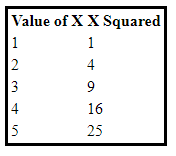# PHP: For Loops

#### Example: Simple for loop

PHP has a very classic syntax for its for loop, simiiar to C. The for loop contains three parts that control how many times the code will loop.

1. \$x=1 creates and assigns 1 to the counter variable
2. \$x <=4 is the test condition. The loop will run while this condition is true
3. \$x++ is the incrementer. Each time through the loop, the \$x variable will increment by 1.
``````
<?php
for (\$x=1; \$x <= 4; \$x++)
{
echo "<p>x = \$x</p>";
}
?>
``````

Run it now

#### Example: Simple for loop - decrementer (counting down)

``````
<?php
for (\$x=4; \$x >= 1; \$x--)
{
echo "<p>x = \$x</p>";
}
?>
``````

Run it now

#### Example: Loop with nested If statement

``````
<?php
for (\$x = 1; \$x <= 6; \$x++)
{
if (\$x == \$answer)
echo "<p>That is the right answer.</p>";
else
echo "<p>That is the wrong answer.</p>";
}
?>
``````

Run it now

Output:

That is the wrong answer.

That is the wrong answer.

That is the wrong answer.

That is the wrong answer.

That is the wrong answer.

That is the right answer.

#### Example: Loop with Math

``````
<?php
\$y = 1;
while (\$x < 6)
{
\$y = \$y + 2;
echo "<p>x equals \$x, y equals \$y.</p>";
\$x++;
}
?>
``````

x equals 1, y equals 3.
x equals 2, y equals 5.
x equals 3, y equals 7.
x equals 4, y equals 9.
x equals 5, y equals 11.

#### Example: Loop to scan for even/odd numbers

``````
<?php
for (\$x=1; \$x < 10; \$x++)
{
\$result = \$x % 2;         // % calculated modulus/mod
if (\$result == 0)
echo "<p>\$x is an even number.</p>";
else
echo "<p>\$x is an odd number.</p>";
}
?>
``````

1 is an odd number.
2 is an even number.
3 is an odd number.
4 is an even number.
5 is an odd number.
6 is an even number.
7 is an odd number.
8 is an even number.
9 is an odd number.

#### Example: Loop with an HTML Table

``````
<?php
echo "<table style=\"border: solid;\">";
echo "<tr><th>Value of X</th><th>X Squared</th></tr>";
for (\$x=1; \$x <= 5; \$x++)
{
echo "<tr>";
echo "<td>\$x</td>";
echo "<td>" . \$x*\$x . "</td>";
echo "</tr>";
}
echo "</table>";
?>
``````

Run it now

Output